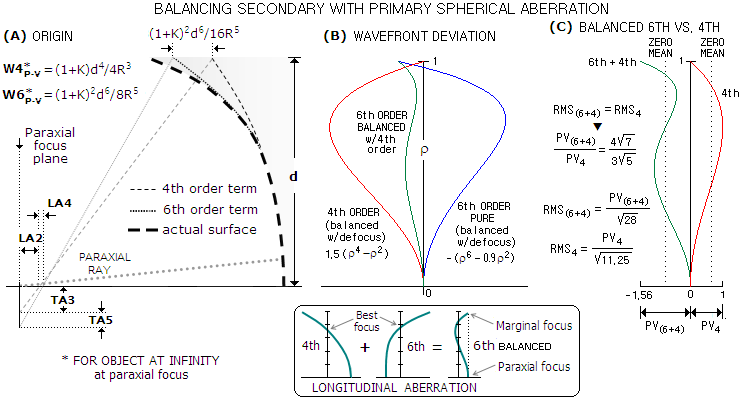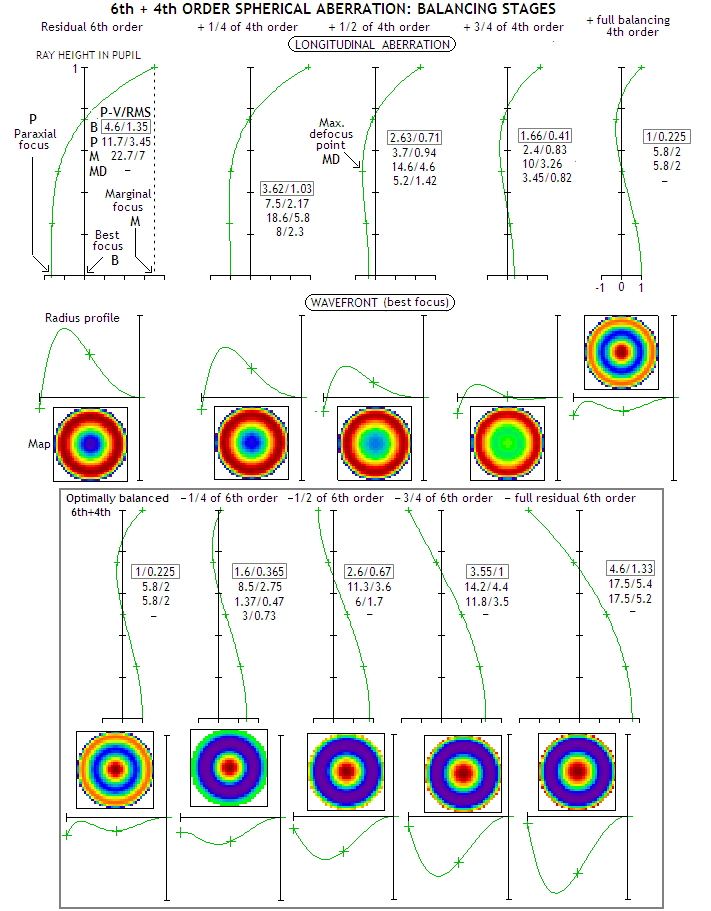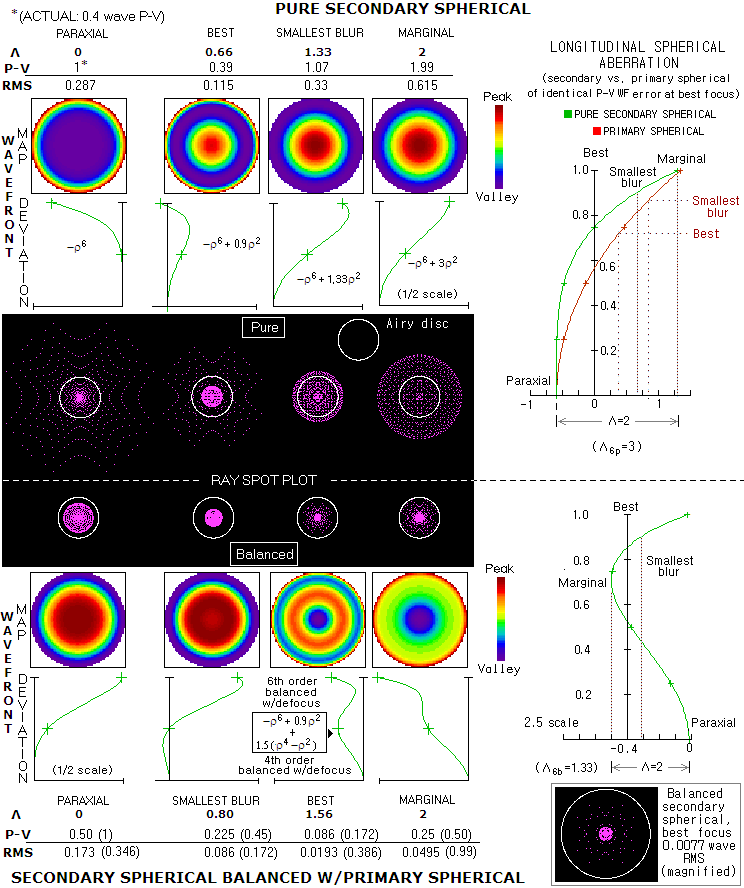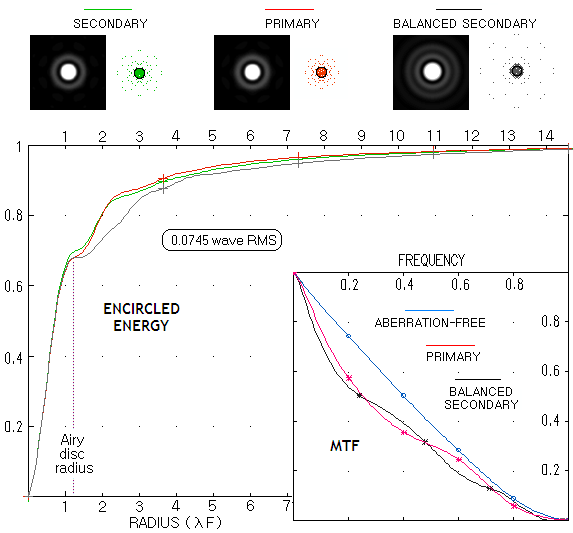telescopeѲptics.net          ▪▪▪▪                                             CONTENTS

# 4.1.3. Higher-order spherical aberration

Considering the importance of center field correction, what is commonly called higher-order - and more specifically secondary spherical aberration, being a result of the second term in the conic surface equation - does have some significance in certain types of amateur telescopes with typically more strongly curved optical surfaces. In particular, in most apochromatic refractors, as well as Maksutov-Cassegrain (MCT) and Schmidt-Cassegrain (SCT) telescopes. Since the Schmidt corrector can directly compensate for higher-order terms as well, they are not a factor in the properly made SCT.

Putting Schmidt-type aspheric on one or more surfaces of an apochromat or MCT is not a practical option; they also generate significantly more of higher-order spherical aberration than a comparable SCT. Higher-order spherical in the MCT can be very effectively lowered by moderately aspherizing primary mirror, which allows to relax corrector's radii. However, all-spherical MCT, as well as apochromats, may have significant level of residual higher-order spherical aberration - which is referred to either as 5th order transverse (ray), or 6th order on the wavefront. It has to be minimized by balancing it with a similar amount of lower-order spherical aberration and defocus. This is usually accomplished by adjusting surface curvature radii, so that they induce needed amount of the lower order aberration. Near-optimum reduction of the 6th order aberration P-V wavefront error by balancing is by a factor of ~0.23, with the PV-to-RMS wavefront error ratio also reduced, by a factor 0.64. In all, the RMS error is reduced approximately by a factor of 0.15 (FIG. 37, B).

Higher-order spherical aberration is merely a consequence of using (close) approximation of the conic surface, instead of the actual surface, in calculating the aberration. General conic surface is described by an expansion series, determining its sagitta (depth at the center) as

z=(d2/2R)+[(1+K)d4/8R3]+[(1+K)2d6/16R5]+... (Eq. 4.6),

with d being the surface half-diameter, R the radius of curvature and K the conic constant. The first term alone accurately describes paraboloid (K=-1) which, consequently, doesn't have higher-order aberration components. The second term describes surface approximations for K≠-1, producing lower-order (3rd order transverse ray, 4th order on the wavefront) spherical aberration for object at infinity (and any object location except that coinciding with the conic surface's geometric focus); the third term in the series adds a correction to that surface approximation, and with it an additional, relatively small amount of the aberration, the already mentioned 5th order transverse, or 6th order on the wavefront.

The order designation is based on the sum of exponents in surface half-diameter d and field angle α. Since spherical aberration doesn't change with the field angle, the exponent in α is zero, and the second term in the expansion series for the surface - and thus for the wavefront as well - is of 4th order; since the exponent in d for the transverse geometric aberration resulting from the 4th order surface approximation is 3, it is a 3rd order transverse aberration.

Terms beyond third in the expansion series are insignificant in amateur-size telescopes.FIGURE 37 TOP: (A) - Higher-order spherical aberrations of reflecting (shown) or refracting conic surface result from corrections to the lower-order surface approximation. The higher-order term that can be significant in amateur telescopes is following the lower-order term in the expansion series. According to the power to the zonal height in its expression, it is referred to either as 5th order transverse ray aberration term, or 6th order on the wavefront for the transverse and wavefront aberration form, respectively (by the same token, longitudinal aberration is 2nd order in its lower-order form, and 4th order in the next higher-form). In the presence of the lower-order aberration on a single surface, it adds to both, its ray (LA=longitudinal aberration, and TA=transverse aberration, shown for the paraxial focus plane) and wavefront error (W). With the lower-order aberration cancelled, it remains as a residual surface aberration.
(B)
- The form of wavefront deviation for pure secondary spherical at the best focus is very similar to that for the primary aberration (the wavefront graph is rotated 90° clockwise to put it in the same orientation as the LA graph). Its function is
ρ6-0.9ρ2, which for its first derivative 6ρ5-1.8ρ (i.e. 6ρ4-1.8 after reduced by ρ) set to zero gives the value of ρ with the maximum deviation as ρ=0.30.25=0.74. When significant, this higher order residual needs to be minimized by correcting the surfaces in their lower-order approximation so that they better approximate the actual surface profile. This is typically the case in telescopes using strongly curved surfaces, such as meniscus corrector in Maksutov-Cassegrain, or lens objective in apochromatic refractors. Secondary spherical can be either removed by applying aspheric term on a surface, or minimized - i.e. "balanced" - with primary spherical aberration, keeping surfaces spherical (note that the relevant form in balancingfor both aberrations is the one balanced with defocus, i.e. best focus aberration). Balancing can be described as "adding" similar amount of lower-order aberration of opposite sign to that of the higher-order. Specifically, the P-V wavefront error of the balancing primary spherical is 0.885 of the P-V wavefront error of secondary spherical, both at their respective best focus location. Balancing is usually accomplished by an appropriate change in the radius of one or more surfaces. Since the two forms of deviations are only similar, not identical, the aberration cannot be cancelled out, but is significantly reduced, by a factor of ~0.15 in the RMS wavefront error. Graph at left shows minimizing 6th order spherical residual in a wider context, starting with the error at paraxial focus. The first two terms in the relation for the balanced 6th/4th order aberration are a sum of the respective errors at the paraxial focus, i.e WFE at that location. The third, defocus term, represents the shift to the best focus location.
(C)
- The minimized wavefront deviation form (expanded in the width for clarity) has an additional curl toward the edge. Compared to primary spherical at best focus, it has greater deviation above 91% of the radius, and below 64%, but the overall deviation is smaller due to its smaller deviation over the 64-91% radius section. Since the P-V/RMS ratio of an aberration is given by a sum of the (absolute) values of its Zernike aberration term for the two values of
ρ corresponding to the maximum wavefront deviation from zero mean and unit coefficient (znm=1) value, it is here given by a sum of absolute values of 7(20ρ6-30ρ4+12ρ2-1)z60 for ρ=0 and ρ=1 (as can be seen above, under C), i.e. 27. Consequently, for given P-V wavefront error, the RMS wavefront error for balanced 4th and 6th order spherical aberration, given by RMSB=P-VB/28, i.e. the RMS error is smaller by a factor of ~0.63 than for primary spherical (and slightly larger for pure secondary spherical) aberration). Note that this balanced form of spherical aberration is often called simply "higher (5th or 6th) order" spherical, which may be confused with the higher-order spherical directly related to the surface term. For this reason, it is better to use the term "balanced 6th/4th-order spherical" for the former.
BOTTOM: As with spherochromatism in general, only a single wavelength can be fully optimized, or in the case of balancing 6th and 4th order aberration optimally balan
ced, i.e. fully minimized. Other wavelengths will be either balanced only partially, or will have the two aberrations add up (being similar in form, when adding up, the sum is roughly arithmetic). Below are illustrated stages of partially balanced 6th order spherical for both, longitudinal aberration (LA) and the corresponding wavefront.The top shows five stages of balancing, starting with pure secondary spherical aberration and adding one quarter of the full primary spherical needed for optimum balancing at the time. Bottom inset starts with the fully balanced form and deducts one quarter of the full amount of 6th order spherical aberration in it, ending with the full balancing 4th order alone (generated by OSLO). Thus, top shows the full amount of balancing higher order with various amounts of primary spherical, and the inset shows the full amount of balancing spherical with various amounts of higher-order spherical. The number columns show the corresponding P-V and RMS wavefront error (WFE) for the P-V error of the fully balanced aberration normalized to unity, for best (B), paraxial (P), marginal (M) and the point of maximum defocus (MD, with respect to paraxial focus, when different from the marginal focus), from top to bottom. Since the two forms are generally similar, but somewhat different, the LA graphs and wavefront shapes for partial balancing are also somewhat different when the 6th order aberration is dominant (top) and when the 4th order is dominant (bottom inset). However for the same numerical proportion of one and the other one, the best focus error and the extent of LA remain at a similar level (comparable mixed aberrations are starting from either full order toward the optimally balanced form, e.g. second from left on top to the forth in the inset). The only significant difference is that the error is the largest at the marginal focus for the former, and at the paraxial focus for the latter. Since the reduction rate at the optimum balance is by a factor of 4.6, 6 and 3.9 for the P-V WFE, RMS WFE and LA extent, the relation between the LA and P-V WFE, given by LA/64F2 (exact for the 4th order and nearly exact for the 6th order), gradually transforms to approx. LA/76F2 at the optimum balance, with the RMS error smaller by a factor of 4.44. The rate of change is somewhat slower up to the level when one of the aberrations is less than a half of the other one, increasing after that. For the points outside the LA range, the P-V WFE error increases roughly by the amount of defocus l from the paraxial or marginal focus, given by W=l/8F2. Note that the specific full magnitude of 6th order aberration here is 0.28 waves P-V, hence with the optimally balanced 6th+4th order of 0.06 wave P-V. Note that OSLO somewhat deviates from the values implied by the theory - 4.3 times smaller P-V and 6.7 times smaller RMS for the minimized vs. pure 6th order spherical - giving somewhat larger reduction in the P-V, but smaller in the RMS error; the difference probably comes from the best focus criterion used by the software.

For object at infinity, reflecting surface forming a perfect wavefront is the paraboloid. Since surface error doubles in the wavefront for paraxial focus, the lower-order P-V wavefront error with respect to the reference sphere centered at paraxial focus is given by double the second term, or W4=(1+K)(ρd)4/4R3. Likewise, the remaining 6th order P-V wavefront aberration is W6=(1+K)2(ρd)6/8R5, with ρ being the height in pupil normalized to 1 and d the pupil (aperture) radius. At the best focus, as mentioned, the P-V wavefront error for the 4th order spherical aberration is smaller by a factor of 0.25, as given by the respective aberration functions, ρ4 for ρ=1 and ρ42 for ρ=0.51/2, for paraxial and best focus, respectively. Due to somewhat different form of deviation, best focus P-V error for the 6th order aberration (balanced with defocus) is reduced somewhat less, by a factor of ~0.4, corresponding to its aberration function for paraxial (ρ6 for ρ=1) and best focus location (ρ6-0.9ρ2 for ρ=0.74); location of the best focus is also shifted from midway between the marginal and paraxial foci to somewhat closer to paraxial focus.

When 6th order spherical aberration is minimized by combining with the lower-order form, the resulting aberration - the 6th/4th order spherical - is also balanced by a specific amount of defocus needed for the shift from paraxial focus to the location with the highest peak diffraction intensity. In other words, it is balanced by both, the lower-order form and defocus (this balancing should not be confused by balancing of the 6th order spherical alone, which is only done by defocusing). As a result, the aberration function changes from

WS6=S6ρ6     and     WS6=S6(ρ6-0.9ρ2)

for the P-V wavefront error of pure 6th order spherical aberration at paraxial and best focus, respectively, where S6 is the peak aberration coefficient for 6th order (secondary) spherical aberration S6=(1+K)2d6/8R5, to

WS(6/4)=S6(ρ6-1.4+0.2-0.05)

for the peak wavefront error (1/2 the P-V error) of balanced 6th/4th order spherical aberration. The term in ρ4 is for balancing with lower-order spherical, and the term in ρ2 for balancing with defocus. The constant term quantifies the relative peak aberration for ρ=1 or ρ=0, which are identical in magnitude, but of opposite sign (as illustrated on FIG. 37, C). Compared to the relative P-V wavefront error of pure (unbalanced, i.e. at the paraxial focus) 6th order spherical (1 for ρ=1), the fully balanced form's P-V error is smaller by a factor of 10; and it is smaller than the pure 6th order balanced with defocus by a factor of 4.3.

The longitudinal 5th order spherical - which is, strictly, 4th order, being proportional to the 4th power of pupil radius - is given by LA5=(K+1)(K+3)(ρd)4/16R3, and adds to the 3rd order aberration, LA3=(K+1)(ρd)2/4R. For K≠1, it is smaller than the lower-order aberration by a factor of (K+3)(ρd)2/4R2.

The transverse 5th order spherical aberration for reflecting conic surface, as the difference between height of marginal ray reflected from 3rd and 5th order surface approximation in the paraxial focus plane (FIG. 37, A) is given by TA5=(K+1)(K+3)(ρd)5/8R4. Hence, it is smaller than 3rd order transverse aberration, whose blur radius is TA3=(K+1)(ρd)3/2R2, by a factor of (3+K)/64F2 (note that this applies for K≠-1). The total transverse aberration is a sum of the two terms, which are of identical sign. Looking at the wavefront-error-to-transverse-aberration ratio, we see that the one for the lower-order aberration is independent of the conic, with the paraxial focus transverse error being larger than the wavefront error by a factor of 16F.

For the higher-order aberration, the wavefront error and transverse aberration doesn't scale evenly with the conic. This means that the aberration changes its form somewhat with the conic, with the paraxial transverse aberration being greater than the wavefront error approximately (due to the best focus wavefront error varying slightly with respect to the error at paraxial focus) by a factor of 16(K+3)F/3(K+1), again, for K≠-1. Given P-V wavefront error, the 5th order transverse paraxial blur for spherical reflecting surface (K=0) is, as expected due to the similar form of wavefront deformation, nearly identical to the lower-order aberration paraxial blur; the corresponding RMS wavefront error is only slightly lower in the former. Best focus blur, being located closer to the paraxial focus, is somewhat larger for the 5th order aberration.

The best focus transverse blur for given P-V wavefront error of balanced 4th and 6th order spherical aberration is larger than the 3rd order blur, mainly due to the strong deviation at the edge of the wavefront. It, however, affects relatively small wavefront area. The RMS wavefront error for the balanced 6th order aberration is actually smaller by a factor of ~0.75, due to relatively large portion of the P-V error being caused by the central deformation, relatively small in area (FIG. 37, C). Contrary to the 3rd order aberration, the paraxial blur for balanced 3rd and 5th order aberration is comparable in size to the best focus blur (FIG. 38). This is due to the paraxial and marginal foci nearly coinciding for optimally balanced 5th order aberration (FIG. 37, B, bottom).FIGURE 38
: Characteristics of pure secondary (5th order transverse, 6th order wavefront) spherical aberration (top) and its minimized form when balanced with primary spherical (bottom), the latter being the one usually referred to as 3th order (transverse), or 4th order (wavefront) spherical aberration. Wavefront errors are normalized to 1 at the pure secondary spherical aberration (PSSA) error at paraxial focus (actual error 0.4 wave P-V), and the balanced longitudinal aberration (LA) graph is scaled 2.5 times the arbitrary unit on the PSSA LA graph (note that the location of paraxial focus remains nearly unchanged after balancing, but longitudinal defocus shifts to the opposite side of it). Longitudinal defocus Λ is normalized to 2, with Λ=0 being the location of paraxial focus, and Λ=2 location of the farthest defocus point (it coincides with the marginal focus point for the unbalanced form, and with 0.72 zone focus with the balanced form of aberration). Note that normalizing LA to 2 for pure and balanced secondary spherical is only for the convenience of making it comparable to primary spherical. For given LA, defocus P-V wavefront error is 3 times larger than the s.a. P-V wavefront error at paraxial focus for pure spherical (thus actual
Λ=3), and 1.33 times for the balanced form (Λ=1.33).
PSSA wavefront data show overall similarity with primary spherical, main exception being that the error at the paraxial focus is somewhat smaller, and at the marginal focus somewhat larger relative to the best focus error, than with primary spherical (note that the P-V WF error ratio at the paraxial vs. best focus for pure secondary spherical is 2.33, not 2.56 as implied by OSLO, probably due to less than perfect accuracy, or different criterion in determining the best focus; related to it, it is to expect that the RMS error reduction at the best focus is somewhat greater than implied and, indeed, it is about 3.6 times smaller, rather than nearly 3.5, as implied by raytrace). This extends to the size of corresponding geometrical blurs. The smaller error at paraxial focus, relative to that at the best focus location, means that less of defocus error is needed to balance PSSA itself (analogous to balancing primary spherical with defocus, not to confuse with balancing PSSA with primary spherical and defocus in order to have it minimized). However, since both are proportionally smaller, the aberration function for best focus location is proportional to ρ
62 (it gives the smallest P-V error, with the shape similar to primary spherical, with zero deviation at the center and the edge, with the maximum deviation at ~0.7 zone; however, the smallest RMS error is achieved with slightly less defocus, ρ6-0.9ρ2, and edge deviation of nearly 30% of the maximum peak deviation at 0.74 zone, opposite in sign). The PSSA LA is nearly identical to that for primary spherical for given P-V wavefront error at the best focus. Since the secondary aberration function ρ6-0.9ρ2 generates larger maximum deviation than the primary function ρ42, the latter is multiplied by a 1.5 factor of 1.5, i.e. 1.5(ρ42) to bring its P-V to the needed proportion vs. secondary aberration P-V to result in the minimum RMS error (specifically, the primary aberration P-V wavefront error is smaller by a factor of 0.885). Thus the wavefront aberration function for balanced secondary spherical aberration is

ρ
6-0.9ρ2-1.5(ρ42)=ρ6-1.5ρ4+0.6ρ2.

As the plot implies, its maximum is for ρ=1. Setting
first function derivative, 6ρ5-6ρ3+1.2ρ, to zero does not give direct solution for the values of ρ for which the tangent of the function is vertical (points of inflection), but solving it by dividing the derivative by 6ρ and reducing it to quadratic function by dividing it with ρ2 gives the solutions as ρ2=0.5± 0.051/2, i.e,
ρ
1=(0.5-0.051/2)1/2=0.526 and ρ2=(0.5+0.051/2)1/2=0.851.
However, the balanced 5th/3rd (i.e. 4th/6th) order spherical is a distinctly different form of spherical aberration. It is already indicated by the shape of its LA graph, where the paraxial and marginal foci nearly coincide. The wavefront error is nearly six times smaller at the best vs. paraxial focus, the latter having twice the error of the marginal focus. As the numbers show, fully minimizing pure higher order spherical aberration by balancing it with primary spherical, results in 4.6 times smaller P-V, and six times smaller RMS wavefront error. The ray spots are much more even in size, but the best focus spot is significantly larger for given RMS wavefront error: with this specific error (0.0077 wave RMS) best focus spot diameter with primary spherical would have been less than 1/3 of the Airy disc's, which makes it nearly three times smaller. The reason is that the dense central portion of the balanced aberration blur, where most of the rays are (bottom corner, right), is of similar size in both aberration forms, and how wide out are spread remaining 20%, or so, of the rays matters little. The significance of uneven rate of aberration change toward the opposite sides of best focus, indicates that the conventional wave of star testing at nearly equal amounts of defocus either side from best focus will be less reliable than with primary spherical aberration.While the primary and pure secondary spherical aberration at best focus location have very similar effect with respect to image quality, balanced secondary spherical differs more than negligibly even at relatively small error levels. Plots at left show OSLO output for 0.0745 wave RMS wavefront error of these three forms of spherical aberration. Due to its double bright ring, balanced secondary spherical encircles about as much energy at 3λF radius as the other two do at 2λF. It also has somewhat more energy spread out in the wider area beyond this point. The corresponding Strehl is also slightly lower, with encircled energy closer to 0.79. The contrast transfer (shown only for balanced primary and secondary, for clarity) is similar, on the average, except the frequencies close to the resolution threshold, which is lower for the balanced form, the consequence of its steep edge wavefront curl being similar to that of turned edge.

An alternative, generally more effective way of correcting higher order spherical aberration is deforming a smooth conic - usually sphere - or flat surface, into a needed irregular radially symmetrical profile, often referred to as higher-order aspheric. Unlike the balancing, which only minimizes present higher-order term, it can completely cancel out not only secondary (5th order transverse, or 6th order wavefront) spherical aberration, but also yet higher order terms. Example of such higher order aspheric is the Schmidt corrector; it could also be used for higher-order aberration balancing (for instance, overcorrecting it with the appropriate amount of 4th order aberration alone would significantly reduce the 6th order aberration error; in, say, 200mm f/2 Schmidt camera, from 0.83 wave P-V/0.227 wave RMS of 6th order spherical to ~0.19 wave P-V and less than 0.05 wave RMS error of balanced aberration), but it is, of course, inferior to the complete correction.

Higher-order spherical is more of a problem in larger apochromatic refractors and, particularly, in instruments using strongly curved meniscus correctors. As the wavefront error of 6th order aberration increases inversely to the 5th power of surface radius, meniscus corrector can generate enormous amount of higher spherical aberration, that cannot be minimized to an acceptable level. This limits relative apertures of mirrors used in these instruments to ~f/3, or slower, even in moderate to small apertures. An effective - although adding to the expense - solution to this problem is aspherizing the primary, which then requires weaker corrector, with significantly lower higher-order spherical aberration contribution.